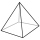# Diagonal + pyramid - math problems

#### Number of problems found: 15

• Quadrilateral pyramidIn a regular quadrilateral pyramid, the side edge is e = 7 dm, and the diagonal of the base is 50 cm. Calculate the pyramid shell area.
• Height of pyramidThe pyramid ABCDV has edge lengths: AB = 4, AV = 7. What is its height?
• Right pyramidA right pyramid on a base 4 cm square has a slant edge of 6 cm. Calculate the volume of the pyramid.
• The pyramid 4sThe pyramid with a rectangular base measuring 6 dm and 8 dm has a side edge of length 13 dm. Calculate the surface area and volume of this pyramid.
• Base diagonalIn a regular 4-sided pyramid, the side edge forms an angle of 55° with the base's diagonal. The length of the side edge is eight meters. Calculate the surface area and volume of the pyramid.
• Tetrahedral pyramidIt is given a regular tetrahedral pyramid with base edge 6 cm and the height of the pyramid 10 cm. Calculate the length of its side edges.
• Square pyramidCalculate the pyramid's volume with the side 5cm long and with a square base, side-base has an angle of 60 degrees.
• Side edgesThe regular 4-sided pyramid has a body height of 2 dm, and the opposite side edges form an angle of 70°. Calculate the surface area and volume of the pyramid.
• Tetrahedral pyramidCalculate the regular tetrahedral pyramid's volume and surface if the content area of the base is 20 cm2, and the deviation angle of the side edges from the plane of the base is 60 degrees.
• Quadrilateral pyramidThe regular quadrilateral pyramid has a base edge a = 1.56 dm and a height h = 2.05 dm. Calculate: a) the deviation angle of the sidewall plane from the base plane b) deviation angle of the side edge from the plane of the base
• Quadrangular pyramidCalculate the surface area and volume of a regular quadrangular pyramid: sides of bases (bottom, top): a1 = 18 cm, a2 = 6cm angle α = 60 ° (Angle α is the angle between the sidewall and the base plane.) S =? , V =?
• Quadrilateral pyramidIn a regular quadrilateral pyramid, the height is 6.5 cm and the angle between the base and the side wall is 42°. Calculate the surface area and volume of the body. Round calculations to 1 decimal place.
• Distance of pointsA regular quadrilateral pyramid ABCDV is given, in which edge AB = a = 4 cm and height v = 8 cm. Let S be the center of the CV. Find the distance of points A and S.
• Hexagon rotationA regular hexagon of side 6 cm is rotated through 60° along a line passing through its longest diagonal. What is the volume of the figure thus generated?
• Billiard ballsA layer of ivory billiard balls of radius 6.35 cm is in the form of a square. The balls are arranged so that each ball is tangent to every one adjacent to it. In the spaces between sets of 4 adjacent balls other balls rest, equal in size to the original.

Do you have an interesting mathematical word problem that you can't solve it? Submit a math problem, and we can try to solve it.

We will send a solution to your e-mail address. Solved examples are also published here. Please enter the e-mail correctly and check whether you don't have a full mailbox.

Please do not submit problems from current active competitions such as Mathematical Olympiad, correspondence seminars etc...

Diagonal - math problems. Pyramid Problems.